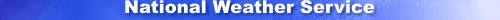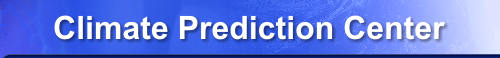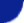Site Map News Organization SearchHOME > Monitoring_and_Data > Oceanic and Atmospheric Data > Reanalysis: Atmospheric Data > wgrib2-new_grid_interpolation

### Introduction

The -new_grid_interpolation option selects the type of interpolation used by the -new_grid option. The possible values are bilinear, bicubic, nearest neighbor, spectral, neighbor-budget and budget. The -new_grid_interpolation option must appear before the -new_grid option. If the -new_grid_interpolation option is not used, the interpolation defaults to bilinear.

1. bilinear, interpolate linearly in X and then linearly in Y
2. bicubic,
3. nearest neighbor, value of closest source grid point in X-Y space
4. budget, make 5x5 grid, find the 25 bilinear values and average
5. neighbor-budget, make 5x5 grid, find 25 nearest neighbor values and average
6. spectral, convert to spectral space (user specified) and then to grid values

Some fields may require different types of interpolation such as the soil type should be found using a nearest neighbor interpolation. (A fractional soil type is meaningless.) It is common practice to use -if and -fi options to control the setting of the the interpolation type as shown by the following command.

```    wgrib2 IN.grb -new_grid_winds earth -new_grid_interpolation bilinear \
-if ":(VGTYP|SOTYP):" -new_grid_interpolation neighbor -fi \
-new_grid latlon 0:360:1 90:181:-1 OUT.grb
```

Budget or neighbor-budget is often used for precipitation in order to roughly conserve global averages. The budget interpolations are slower because the output grid cell is covered with a 5x5 grid, and interpolations are done for each point of the 5x5 grid. So the budget interpolations do 25 times more interpolations.

Spectral interpolation is specialized interpolation scheme. A global field is transformed into spherical harmonics (user specified truncation), and the grid point values are determined from the spherical harmonics. This interpolation is unlike other interpolation scheme. For example, the nearest neighbor is based on the 1 grid point, the bilinear internpolation is based on 4 grid points. The spectral interpolation is based on all the grid points. So the interpolation scheme will act as noise reduction when projected to a fewer spherical harmonics than the number of grid points.

The limiting zonal wave number is specified and should not be prime as it makes the Fast Fourier Transform into a slow Fourier Transform. The limiting zonal wave number should be a product of many 2s, 3s, 5s and other small primes. The limiting zonal wave number may need to be compatible with the Fast Fourier Transform (FFT) code used.

### Usage

```-new_grid_interpolation X
X = bilinear, bicubic, neighbor, budget, neighbor-budget, spectral-(trun)(num)
spectral-*  is alpha

bilinear:          linear interpolation in X and then Y.
neighbor:          Values from nearest grid point.
budget:            Preserves large-scale means. Good for precipitation.
neighbor-budget:   Preserves large-scale means. Good for precipitation.
spectral:          Converts a global field into spectral harmonics
and derives values from the spectral harmonics.
trun = 't', 'T', 'r' or 'R' for triangular or rhomboidal trunction
(num) = max zonal wave number
The input has to be a global field and contain valid values for all grid points.
If undefined values are found, bilinear interpolation is used.
```